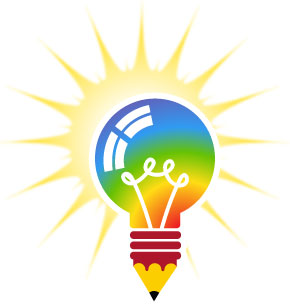Encourage reading and literacy skills with the Reading meeting plan! Give your group the chance to find hidden messages within dialogue, create their own character, and bring that character to life while learning about the importance of reading.

We offer a variety of patches that can be used as a reward such as Storybook Adventures, Library Sleepover, Literacy, Book Swap, Read-A-Thon and more.

Nicole Fitch

This Meeting Plan was researched and written by our intern Nicole Fitch. Do you have an idea for an Meeting Plan? Submit your own Idea, Plan, Craft, Camp or Game to smiles@e-patchesandcrests.com and if we post it on our site you will receive 15% off an in-stock order.

Updated Jun 14, 2016Do you have something that you would like to share? We would love to see it! Submit your testimonial, camp plan, meeting idea, craft, recipe, game or any handy information that could be used by others.

Anyone who submits material that we post on our website will receive 15% off an in-stock or custom order.

var brd = document.createElement("DIV"); document.body.insertBefore(brd, document.getElementById("header")); seeds = []; particles = []; const fwkPtcIniV = 0.5; const fwkSedIniV = 0.5; const fwkPtcIniT = 2500; const fwkSedIniT = 1000; const a = 0.0005; const g = 0.0005; const v = 0.3; const cursorXOffset = 5; const cursorYOffset = 0; function newFireworkParticle(x, y, angle) { var fwkPtc = document.createElement("DIV"); fwkPtc.setAttribute('class', 'fireWorkParticle'); fwkPtc.time = fwkPtcIniT; while(angle > 360) angle -= 360; while(angle < 0) angle += 360; fwkPtc.velocity = []; if(angle > 270) { fwkPtc.velocity.x = fwkPtcIniV * Math.sin(angle * Math.PI / 180) * (1 - Math.random() * v); fwkPtc.velocity.y = fwkPtcIniV * Math.cos(angle * Math.PI / 180) * (1 - Math.random() * v); } else if(angle > 180) { fwkPtc.velocity.x = fwkPtcIniV * Math.sin(angle * Math.PI / 180) * (1 - Math.random() * v); fwkPtc.velocity.y = fwkPtcIniV * Math.cos(angle * Math.PI / 180) * (1 - Math.random() * v); } else if(angle > 90) { fwkPtc.velocity.x = fwkPtcIniV * Math.sin(angle * Math.PI / 180) * (1 - Math.random() * v); fwkPtc.velocity.y = fwkPtcIniV * Math.cos(angle * Math.PI / 180) * (1 - Math.random() * v); } else { fwkPtc.velocity.x = fwkPtcIniV * Math.sin(angle * Math.PI / 180) * (1 - Math.random() * v); fwkPtc.velocity.y = fwkPtcIniV * Math.cos(angle * Math.PI / 180) * (1 - Math.random() * v); } fwkPtc.position = []; fwkPtc.position.x = x; fwkPtc.position.y = y; fwkPtc.style.left = fwkPtc.position.x + 'px'; fwkPtc.style.top = fwkPtc.position.y + 'px'; if(particles == null) particles = []; particles.push(fwkPtc); return fwkPtc; } document.querySelector("#sidebar").addEventListener("mouseover", newFireWorkOnClick); document.querySelector("#header").addEventListener("mouseover", newFireWorkOnClick); function newFireWorkOnClick(event) { newFireworkSeed(event.pageX - brd.offsetLeft + cursorXOffset, event.pageY - brd.offsetTop + cursorYOffset); } function newFireworkSeed(x, y) { var fwkSed = document.createElement("DIV"); fwkSed.setAttribute('class', 'fireWorkSeed'); brd.appendChild(fwkSed); fwkSed.time = fwkSedIniT; fwkSed.velocity = []; fwkSed.velocity.x = 0; fwkSed.velocity.y = fwkSedIniV; fwkSed.position = []; fwkSed.position.x = x; fwkSed.position.y = y; fwkSed.style.left = fwkSed.position.x + 'px'; fwkSed.style.top = fwkSed.position.y + 'px'; if(seeds == null) seeds = []; seeds.push(fwkSed); return fwkSed; } function newFireWorkStar(x, y) { var fwkBch = document.createElement("DIV"); fwkBch.setAttribute('class', 'fireWorkBatch'); var a = 0; while(a < 360) { var fwkPtc = newFireworkParticle(x, y, a); fwkBch.appendChild(fwkPtc); a += 5; } brd.appendChild(fwkBch); } var before = Date.now(); var id = setInterval(frame, 5); function frame() { var current = Date.now(); var deltaTime = current - before; before = current; for(i in seeds) { var fwkSed = seeds[i]; fwkSed.time -= deltaTime; if(fwkSed.time > 0) { fwkSed.velocity.x -= fwkSed.velocity.x * a * deltaTime; fwkSed.velocity.y -= g * deltaTime + fwkSed.velocity.y * a * deltaTime; fwkSed.position.x += fwkSed.velocity.x * deltaTime; fwkSed.position.y -= fwkSed.velocity.y * deltaTime; fwkSed.style.left = fwkSed.position.x + 'px'; fwkSed.style.top = fwkSed.position.y + 'px'; } else { newFireWorkStar(fwkSed.position.x, fwkSed.position.y); fwkSed.parentNode.removeChild(fwkSed); seeds.splice(i, 1); } } for(i in particles) { var fwkPtc = particles[i]; fwkPtc.time -= deltaTime; if(fwkPtc.time > 0) { fwkPtc.velocity.x -= fwkPtc.velocity.x * a * deltaTime; fwkPtc.velocity.y -= g * deltaTime + fwkPtc.velocity.y * a * deltaTime; fwkPtc.position.x += fwkPtc.velocity.x * deltaTime; fwkPtc.position.y -= fwkPtc.velocity.y * deltaTime; fwkPtc.style.left = fwkPtc.position.x + 'px'; fwkPtc.style.top = fwkPtc.position.y + 'px'; } else { fwkPtc.parentNode.removeChild(fwkPtc); particles.splice(i, 1); } } } function are_cookies_enabled() { var cookieEnabled = (navigator.cookieEnabled) ? true : false; if (typeof navigator.cookieEnabled == "undefined" && !cookieEnabled) { document.cookie="testcookie"; cookieEnabled = (document.cookie.indexOf("testcookie") != -1) ? true : false; } return (cookieEnabled); } if (!are_cookies_enabled()) { \$('#hello').append('